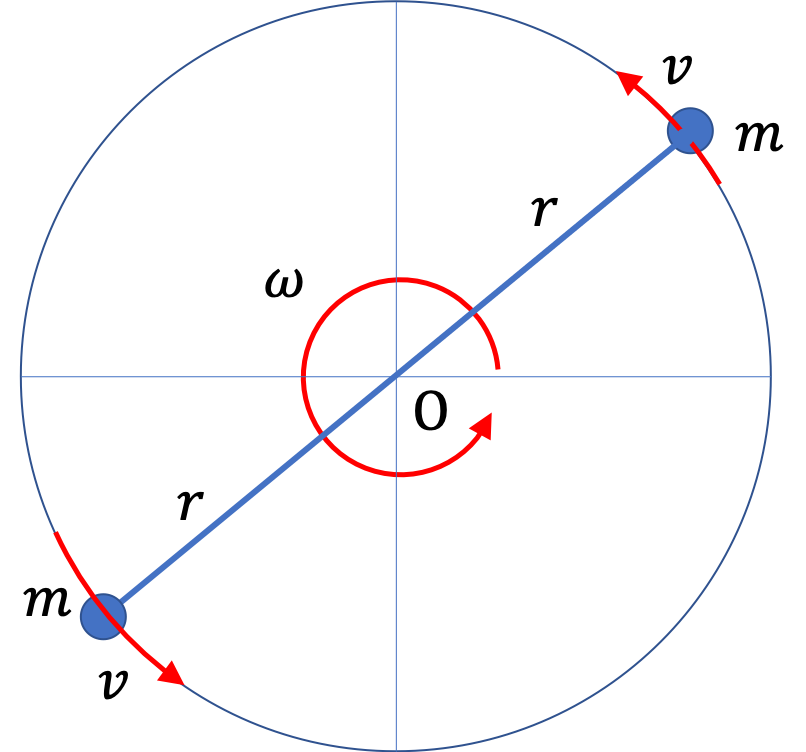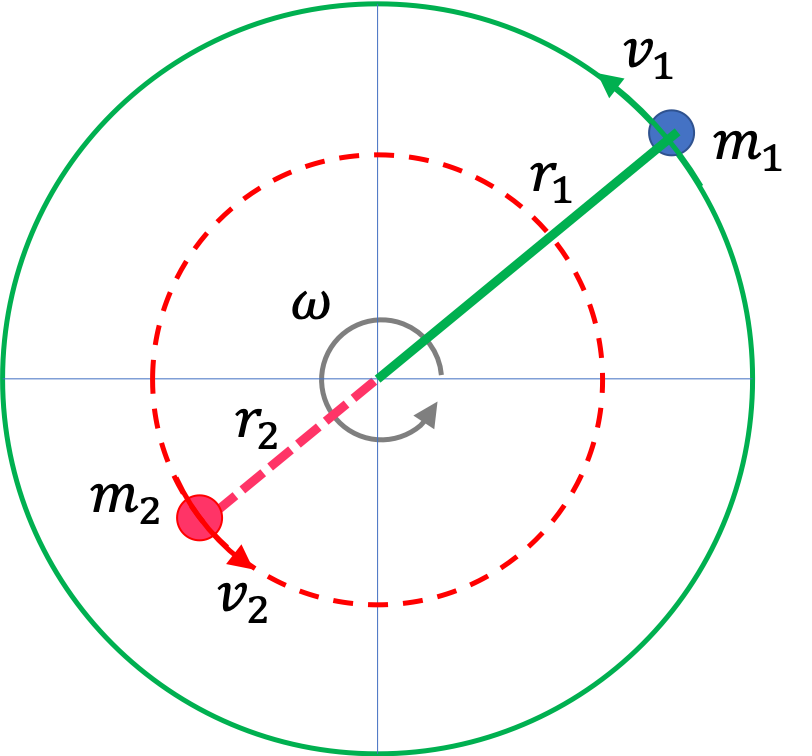## Section8.3Kinetic Energy

Kinetic energy or the motional energy of a particle of mass $m$ moving with speed $v$ is defined by the following formula.

\begin{equation} K = \dfrac{1}{2}\,m\,v^2.\label{eq-kinetic-energy-def-fundamental}\tag{8.3.1} \end{equation}

Sometimes, we will also denote kinetic energy by $\text{KE}\text{.}$ Using momentum $p=mv\text{,}$ we can also write kinetic energy as

\begin{equation*} K = \dfrac{p^2}{2m}. \end{equation*}

The value of KE is frame-dependent. Suppose you are sitting in a train which is moving with respect to the ground. Then, your kinetic energy will be zero with respect to the train train but non-zero with respect to the ground.

An important thing to note about kinetic energy is that it is a scalar quantity - just a number, actually a positive number. If a system contains more than one particle, the total kinetic energy is just an algebraic sum of the parts.

\begin{equation} K = \dfrac{1}{2}\,m_1\,v_1^2 + \dfrac{1}{2}\,m_2\,v_2^2 + \dfrac{1}{2}\,m_3\,v_3^2 + \cdots\label{eq-kinetic-energy-def-n-body}\tag{8.3.2} \end{equation}

If every particle of a body is moving with the same speed, say $v\text{,}$ then, the sum on the right side is trivial.

\begin{equation*} K = \dfrac{1}{2}\,m_1\,v^2 + \dfrac{1}{2}\,m_2\,v^2 + \dfrac{1}{2}\,m_3\,v^2 + \cdots = \ \dfrac{1}{2}\,M\, v^2, \end{equation*}

where $M = \left( \,m_1 + \,m_2 + \,m_3 + \cdots\, \right) \text{,}$ the total mass. This would be the case for a rigid body in a pure translation motion.

The unit of kinetic energy can be obtained by multiplying the units of mass and velocity squared. In SI system, the result is named Joule and abbreviated $\text{J} \text{.}$

\begin{equation*} \text{J} = \text{kg.m}^2\text{/s}^2 \end{equation*}

### Subsection8.3.1Kinetic Energy for Circular Motion

Often we encounter situations in which a mass is moving in a circle. In these cases, we can write speed $v$ in terms of angular speed $\omega$ and radius $R$ of the circle.

\begin{equation*} v = R \omega. \end{equation*}

Therefore, kinetic energy will have alternate expression.

\begin{equation} K = \frac{1}{2} m v^2 = \frac{1}{2} m R^2 \omega^2. \tag{8.3.3} \end{equation}

When an object is going in a circle, we also say that it is rotating about an axis through the center of the circle. In the language of rotation the quantity multiplying $\frac{1}{2}\omega^2$ in the formula for kinetic energy, is also called moment of inertia. Moment of inertia is usually denoted by letter $I\text{.}$

\begin{equation*} I = m R^2. \end{equation*}

Therefore, when a point mass $m$ is moving in a circle with angular speed $\omega\text{,}$ its kinetic energy formula can also be written as

\begin{equation*} K = \frac{1}{2} I \omega^2, \end{equation*}

which has a nice analogy to the $\frac{1}{2}mv^2$ with $I$ replacing $m$ and $\omega$ replacing $v\text{.}$

A baton has two balls at the end of a light beam. Let the masses of the two balls be $0.25\text{ kg}$ each. The baton is gently dropped from some height so that, after some time, the two balls have the same speed of $10\text{ m/s} \text{.}$ What is the kinetic energy of the baton at this instant? Ignore the mass of the beam.Hint

$\dfrac{1}{2}Mv^2 \text{.}$

$25\text{ kg.m}^2\text{/s}^2.$

Solution

Since the two masses are moving at the same speed, we can just add up the masses and apply one simple formula.

\begin{align*} K \amp = \dfrac{1}{2}m_1v^2 + \dfrac{1}{2}m_2v^2 = \dfrac{1}{2}(m_1 + m_2)v^2\\ \amp = \dfrac{1}{2} 0.5\times 10^2 = 25 \text{ kg.m}^2\text{/s}^2 = 25\text{ J}. \end{align*}

A baton has two balls at the end of a light beam. Let the masses of the two balls be $0.25\text{ kg}$ each. The baton is throw such that at a later time the baton is tumbling such that at a particular instant, one of the balls has a speed of $10\text{ m/s}$ and the other has the speed of $16\text{ m/s} \text{.}$ What is the kinetic energy of the baton? Ignore the mass of the beam.

Hint

Use $\dfrac{1}{2} m_1 v_1^2 + \dfrac{1}{2} m_2 v_2^2\text{.}$

$44.5\text{ J}.$

Solution

Since the two masses are moving at different speeds, we will have to work out the kinetic energies of the two balls separately and then add them up.

\begin{align*} K \amp = \dfrac{1}{2} m_1 v_1^2 + \dfrac{1}{2} m_2 v_2^2 \\ \amp = \dfrac{1}{2} m ( v_1^2 + v_2^2 )\\\ \amp = \dfrac{1}{2} \times 0.25 \times ( 10^2 + 16^2 ) = 44.5 \text{ J}. \end{align*}

A train is moving at speed $3\text{ m/s}$ towards West. Inside the train, a toy car of mass $0.2\text{ kg}$ is moving with speed $4\text{ m/s}$with respect to the train due North. (a) What is the kinetic energy of the toy with respect to the train? (b) What is the kinetic energy of the toy with respect to the ground?

Hint

(a) Use the given speed. (b) Add velocities to find the velocity of the toy with respect to the ground. Find speed from this velocity.

(a) $1.6\text{ J}\text{,}$ (b) $2.5\text{ J}\text{.}$

Solution 1 (a)

Since speed with respect to train is already given in the problem, its easy.

\begin{equation*} K = \frac{1}{2}\times 0.2 \times 4^2 = 1.6\text{ J}. \end{equation*}
Solution 2 (b)

We need to find the speed with respect to the ground from the addition of the two velocities.

\begin{equation*} \vec v_\text{of car wrt ground} = \vec v_\text{of train wrt ground} + \vec v_\text{of car wrt train}. \end{equation*}

The magnitude of this velocity is needed for th eKE formula. This is a vector equation. Let West be positive $x$ and North be positive $y$ directions. Then, we get

\begin{equation*} \vec v_\text{of car wrt ground} = 3\,\hat i + 4\, \hat j. \end{equation*}

Therefore, speed of interest will be

\begin{equation*} v = \sqrt{3^2 + 4^2} = 5\text{ m/s}. \end{equation*}

Therefore, kinetic energy of the toy with respect to ground will be

\begin{equation*} K = \frac{1}{2}\times 0.2 \times 5^2 = 2.5\text{ J}. \end{equation*}

A baton has two balls at the end of a light beam of length $0.80\text{ m} \text{.}$ Let the masses of the two balls be $0.25\text{ kg}$ each. The baton is gently held at the center and rotated at an angular speed of $10\text{ rad/sec} \text{.}$ What is the kinetic energy of the baton? Ignore the mass of the beam.Hint

Use $I = m_1r_1^2 + m_2r_2^2\text{.}$

$4.0\text{ J}.$

Solution

Since the baton is in a pure rotation, we wil use the kinetic energy formula for the pure rotation.

\begin{align*} K \amp = \dfrac{1}{2}\, I\, \omega^2, \\ \amp = \dfrac{1}{2} (m_1r_1^2 + m_2r_2^2)\,\omega^2,\\ \amp = \dfrac{1}{2}\, 2\, m\, r^2\, \omega^2, \\ \amp = 0.25 \times 0.4^2\times 10^2 = 4.0 \text{ J}. \end{align*}

A baton has two balls at the end of a light beam of length $0.80\text{ m} \text{.}$ Let the masses of the two balls be $0.25\text{ kg}$ each. The baton is gently held $0.20\text{ m}$ away from the center and rotated at an angular speed of $10\text{ rad/sec} \text{.}$ In a pure rotation, all parts of a rigid body have the same angular speed. What is the kinetic energy of the baton? Ignore the mass of the beam.Hint

Use $I = m_1r_1^2 + m_2r_2^2\text{.}$

$5.0\text{ J}.$